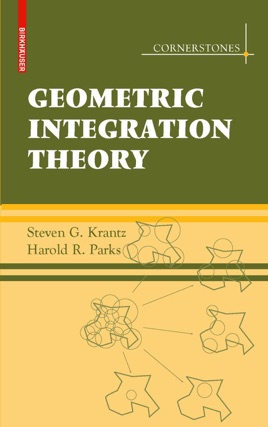• 49,99 €

## Description de l’éditeur

This textbook introduces geometric measure theory through the notion of currents. Currents—continuous linear functionals on spaces of differential forms—are a natural language in which to formulate various types of extremal problems arising in geometry, and can be used to study generalized versions of the Plateau problem and related questions in geometric analysis.

Key features of Geometric Integration Theory:

* Includes topics on the deformation theorem, the area and coarea formulas, the compactness theorem, the slicing theorem and applications to minimal surfaces

* Applies techniques to complex geometry, partial differential equations, harmonic analysis, differential geometry, and many other parts of mathematics

* Provides considerable background material for the student

Motivating key ideas with examples and figures, Geometric Integration Theory is a comprehensive introduction ideal for use in the classroom and for self-study. The exposition demands minimal background, is self-contained and accessible, and thus is ideal for graduate students and researchers.